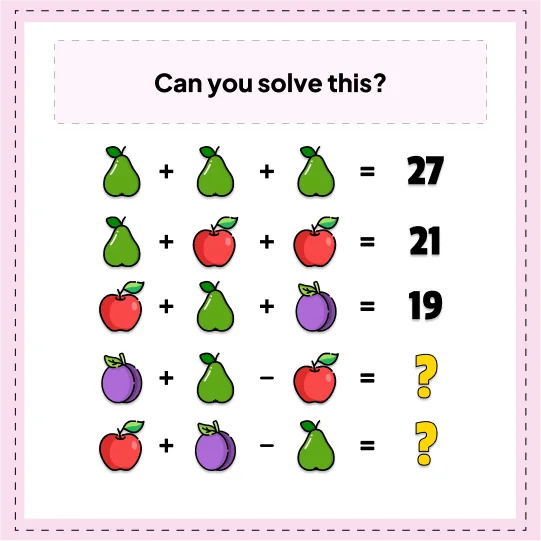# Equation photo- Math Riddle

###### 88.Math Riddles
`Can you solve this? `•

```Explanation :

You see, sometimes it's necessary to compare apples and bananas. Or, in this case,
it could be pears instead of bananas.
Pear = 9, apple = 6, plum = 4 and these make the answers 7 and 1 respectively.
so 4+9+6 =19.ers 7 and 1 respectively.```